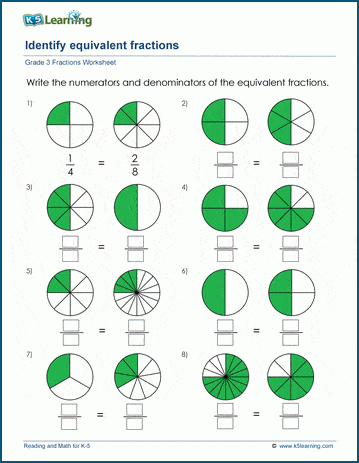# Identify equivalent fractions

## Worksheets: identifying equivalent fractions using pie charts

Below are six versions of our grade 3 math worksheet on identifying equivalent fractions using pie charts; students are shown sets of two pie charts representing equivalent fractions and are asked to write numerators and denominators for each fraction. These worksheets are pdf files.## More fractions worksheets

Explore all of our fractions worksheets, from dividing shapes into "equal parts" to multiplying and dividing improper fractions and mixed numbers.

## What is K5?

K5 Learning offers reading and math worksheets, workbooks and an online reading and math program for kids in kindergarten to grade 5.  We help your children build good study habits and excel in school.